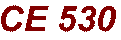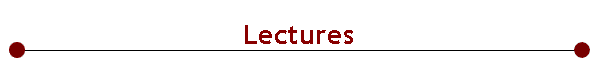Material from class lectures is placed here. Pdf versions contain corrections and updates that are not included in the html version. Lecture 1 [pdf].  Introduction Lecture 2 [pdf].  Properties, units & dimensions; hard-sphere MD Lecture 3 [pdf].  Periodic boundaries, simulation structure, confidence limits, initialization Lecture 4 [pdf].  Object oriented programming, the molecular simulation API. Lecture 5.  Study of some the Java source code. Lecture 6 [pdf].  Statistical mechanics Lecture 7 [pdf].  Monte Carlo integration Lecture 8 [pdf].  Markov processes Lecture 9 [pdf].  Monte Carlo simulation Lecture 10 [pdf]. Simple biasing techniques Lecture 11 [pdf]. Introduction to molecular dynamics Lecture 12 [pdf]. Measurement of transport coefficients; time correlation functions Lecture 13 [pdf]. Molecular dynamics at constant temperature and pressure Lecture 14 [pdf]. Molecular modeling Lecture 15 [pdf]. Long-range interactions and Ewald sum Lecture 16 [pdf]. Dielectrics Lecture 17 [pdf]. Beyond atoms:  Simulating molecules Lecture 18 [ppt.pdf] [opt.pdf]. Free-energy calculations Lecture 19. Distribution methods.  Accuracy and precision of FEP calculations Lecture 20 [pdf]. Phase equilibria calculations Lecture 21 [pdf]. Histogram reweighting method Lecture 22 [pdf]. Configurational bias and methods for chain molecules Lecture 23. Symmetry in MD integrators. RESPA. Lecture 24. Non-equilibrium molecular dynamics, linear-response theory Lecture 25. Efficiencies, algorithms for parallel computing platformsThis material is subject to the Copyright Notice on the home page of this site